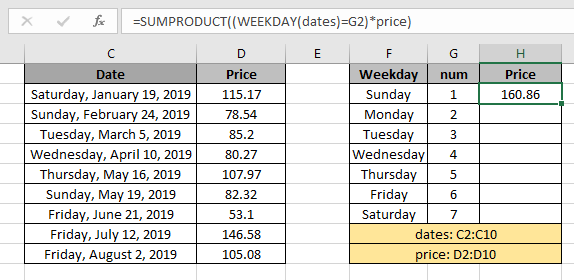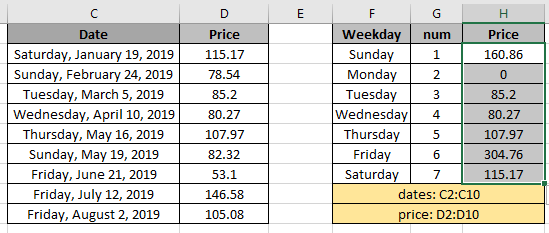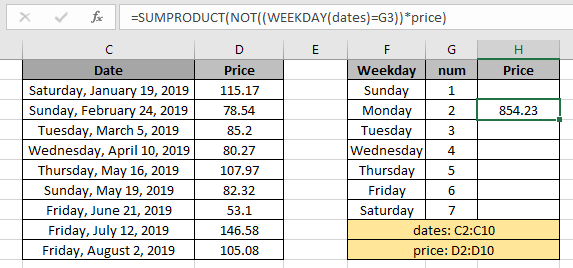# Price SUM by weekdays in Excel

In this article, we will learn to return the price SUM by weekdays in Excel.

Scenario:

In simple words, while working with price filled data. Sometimes, we need to find the sum of price values having a condition. Condition is to get the weekday values considering the given weekday. See the below formula to calculate using the .

How to solve the problem?

For this article we will be needing the use the following functions:

Now we will make a formula out of these functions. Here we will given the data and we needed sum results where weekday is given with weekday_num.

Syntax formula:

= SUMPRODUCT ( ( WEEKDAY ( dates ) = weekday_num ) * price )

Dates: date values provided as cell reference or using the named range.

Weekday_num: number representing weekday of the week.

price : amounts or price values to add.

Example:

All of these might be confusing to understand. So, let's test this formula via running it on the example shown below. Here we have data having Price of products received on the dates. Here we need to find the SUM of price for all 7 weekdays. So for that we have assigned weekday_nums in a column for the formula. Now we will use the below formula to get the SUM of price

Formula:

= SUMPRODUCT ( ( WEEKDAY (dates)=G2)*price)

dates : named range used for the range C2:C10

G2 : cell reference used for the value in G2

price : named range used for the range D2:D10

Explanation:

• Weekday function checks the week_num in G2 as returns TRUE and FALSE for the array of dates.
• SUMPRODUCT function considers value 1 for every TRUE value received and 0 for FALSE value as shown below.

= SUMPRODUCT ( { 0 ; 1 ; 0 ; 0 ; 0 ; 1 ; 0 ; 0 ; 0 } * { 115.17 ; 78.54 ; 85.2 ; 80.27 ; 107.97 ; 82.32 ; 53.1 ; 146.58 ; 105.08   )

• The 1s & 0s in the first array is the result of weekday function and price values in the second array is the named range result.
• After taking product with respective values, The formula drops down to this. The formula adds up the values to get the sum

= SUMPRODUCT ( { 0 ; 78.54 ; 0 ; 0 ; 0 ; 82.32 ; 0 ; 0 ; 0 } )Here the array to the function is given as the named range and cell as cell_reference. Press Enter to get the price results.As you can see in the above snapshot the sum of price values extracted with the weekday_num.

Use of NOT function in the formula:

If you need to find the sum of values not having the specific weekday use the NOT function with the WEEKDAY function.

Use the formula:

= SUMPRODUCT( NOT(( WEEKDAY (dates) = G2 )) * price )

NOT function turns 0s to 1s and 1s to 0s. The rest of the formula is the same so as to get the count not including the given weekend.As you can see from the above formula returns the negation of the weekday value. Here are some observational results using the above formula used.

Notes:

1. The SUMPRODUCT function considers non - numeric values as 0s.
2. The SUMPRODUCT function considers logic value TRUE as 1 and False as 0.
3. The argument array must be of the same length else the function returns the error.

Hope this article about how to SUM price value by weekdays in Excel is explanatory. Find more articles on summing functions here. If you liked our blogs, share it with your fristarts on Facebook. And also you can follow us on Twitter and Facebook. We would love to hear from you, do let us know how we can improve, complement or innovate our work and make it better for you. Write us at info@exceltip.com.

Related Articles

How to use the SUMPRODUCT function in Excel: Returns the SUM after multiplication of values in multiple arrays in excel.

How to use the WEEKDAY function in Excel: Weekday function returns the week number for the given date in excel.

SUM if date is between : Returns the SUM of values between given dates or period in excel.

Sum if date is greater than given date: Returns the SUM of values after the given date or period in excel.

2 Ways to Sum by Month in Excel: Returns the SUM of values within a given specific month in excel.

How to Sum Multiple Columns with Condition: Returns the SUM of values across multiple columns having condition in excel.

Popular Articles

50 Excel Shortcut to Increase Your Productivity : Get faster at your task. These 50 shortcuts will make you work even faster on Excel.

How to use the VLOOKUP Function in Excel : This is one of the most used and popular functions of excel that is used to lookup value from different ranges and sheets.

How to use the COUNTIF function in Excel : Count values with conditions using this amazing function. You don't need to filter your data to count specific values. Countif function is essential to prepare your dashboard.

How to Use SUMIF Function in Excel : This is another dashboard essential function. This helps you sum up values on specific conditions.

Terms and Conditions of use

The applications/code on this site are distributed as is and without warranties or liability. In no event shall the owner of the copyrights, or the authors of the applications/code be liable for any loss of profit, any problems or any damage resulting from the use or evaluation of the applications/code.